Examples

Chapter 6 Class 12 Application of Derivatives
Serial order wise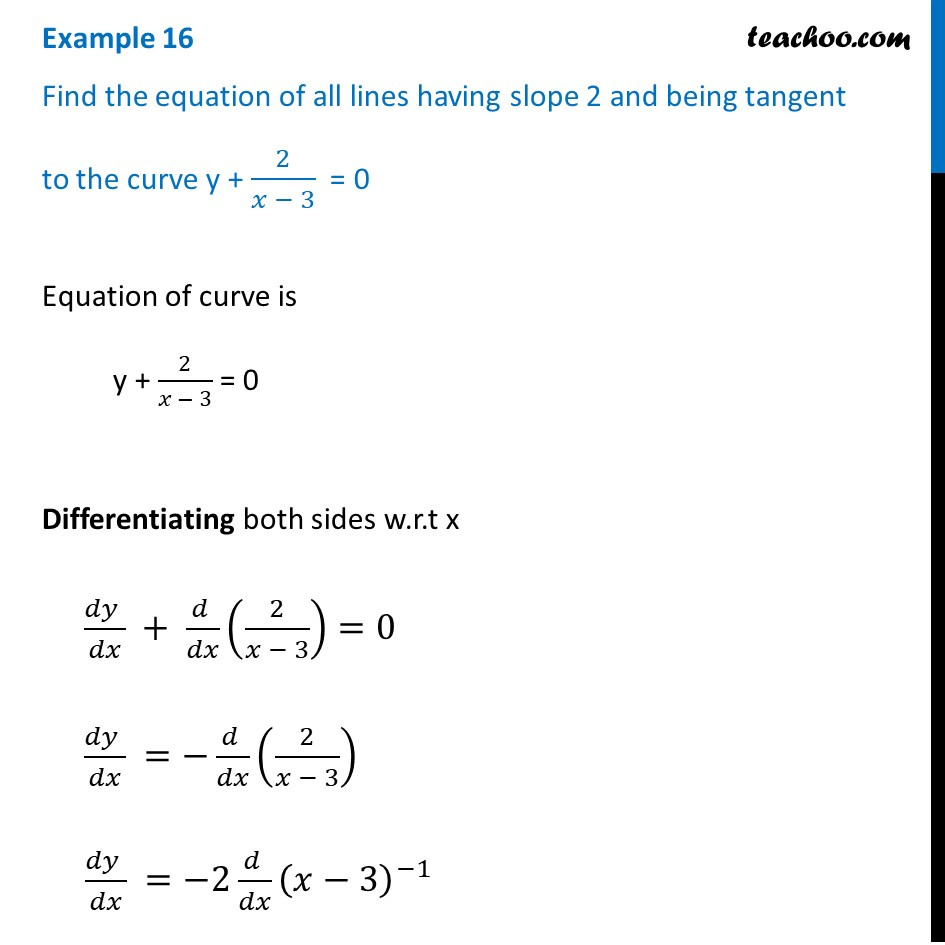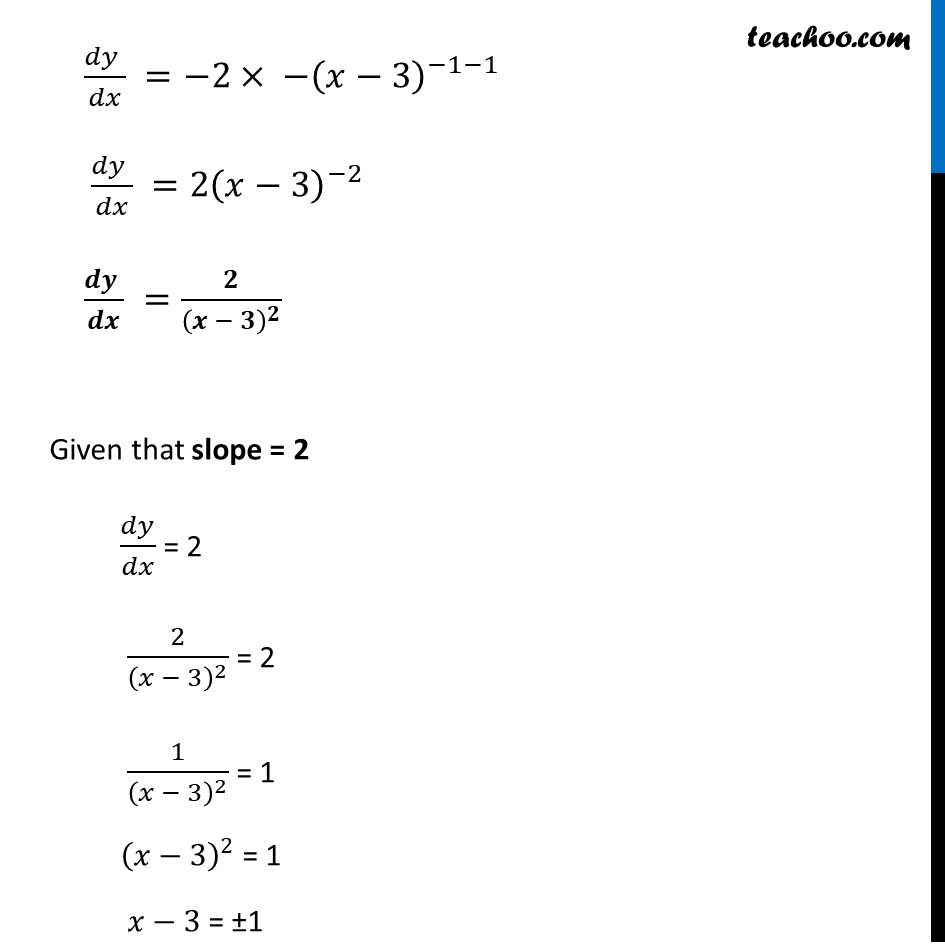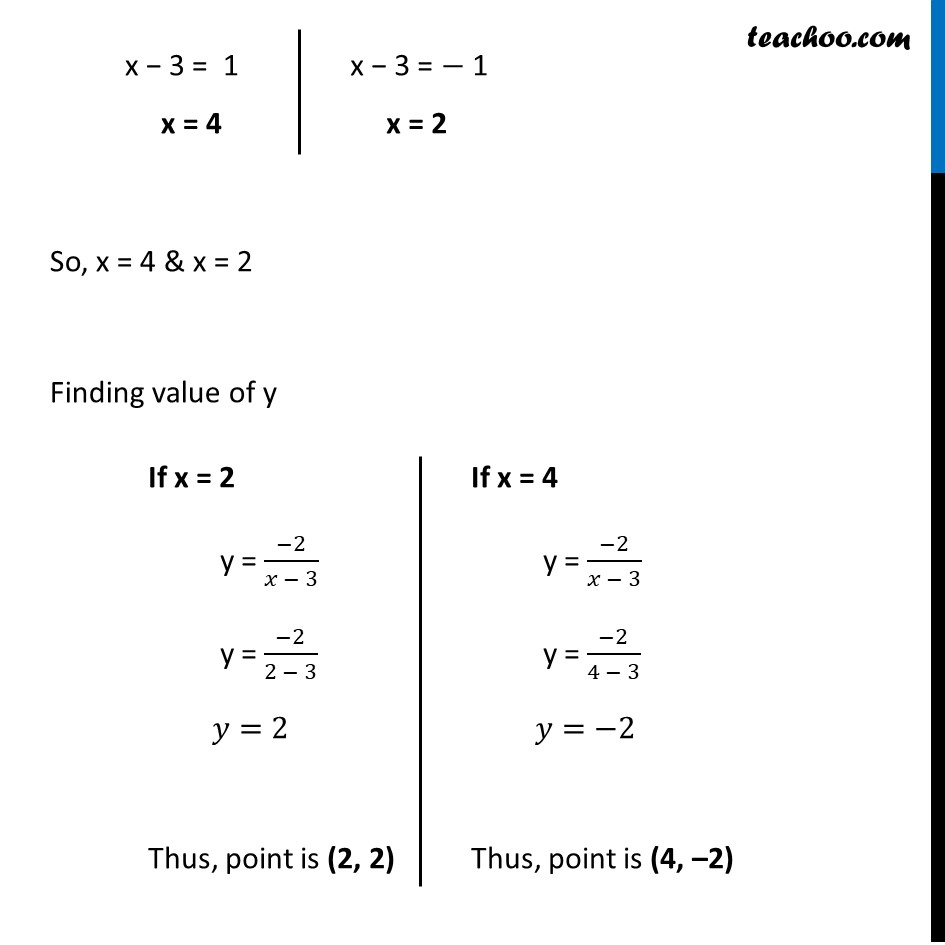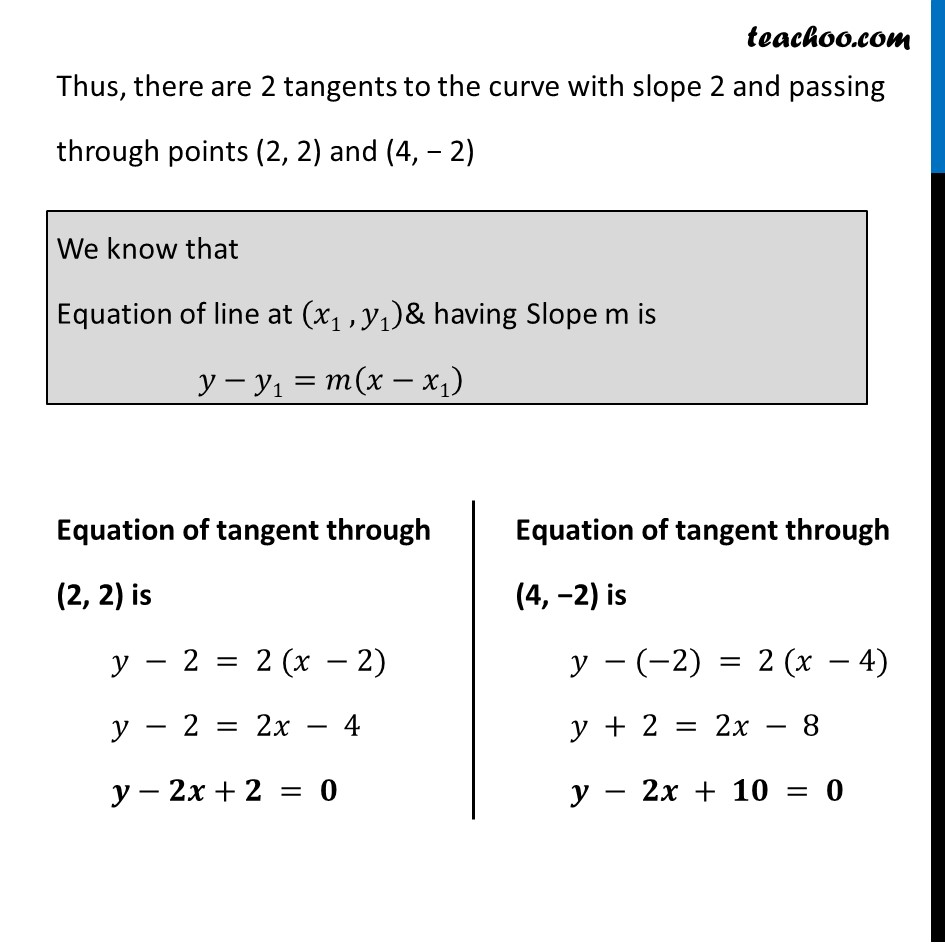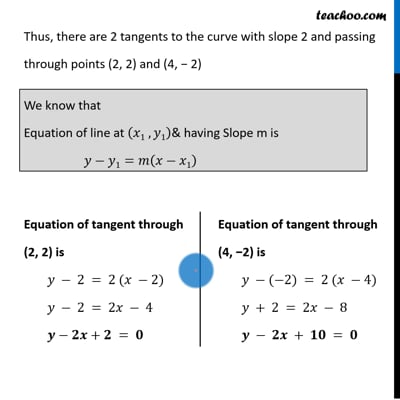This video is only available for Teachoo black users

Solve all your doubts with Teachoo Black (new monthly pack available now!)

### Transcript

Example 16 Find the equation of all lines having slope 2 and being tangent Equation of curve is y + 2/(𝑥 − 3) = 0 Differentiating both sides w.r.t x (𝑑𝑦 )/𝑑𝑥 + (𝑑 )/𝑑𝑥 (2/(𝑥 − 3))=0 (𝑑𝑦 )/𝑑𝑥 =−(𝑑 )/𝑑𝑥 (2/(𝑥 − 3)) (𝑑𝑦 )/𝑑𝑥 =−2 (𝑑 )/𝑑𝑥 (𝑥−3)^(−1) (𝑑𝑦 )/𝑑𝑥 =−2〖 × −(𝑥−3)〗^(−1−1) (𝑑𝑦 )/𝑑𝑥 =2(𝑥−3)^(−2) (𝒅𝒚 )/𝒅𝒙 =𝟐/(𝒙 − 𝟑)^𝟐 Given that slope = 2 𝑑𝑦/𝑑𝑥 = 2 2/(𝑥 − 3)^2 = 2 1/(𝑥 − 3)^2 = 1 (𝑥−3)^2 = 1 𝑥−3 = ±1 x − 3 = 1 x = 4 x − 3 = − 1 x = 2 x − 3 = − 1 x = 2 So, x = 4 & x = 2 Finding value of y If x = 2 y = (−2)/(𝑥 − 3) y = (−2)/(2 − 3) 𝑦=2 Thus, point is (2, 2) If x = 4 y = (−2)/(𝑥 − 3) y = (−2)/(4 − 3) 𝑦=−2 Thus, point is (4, –2) Thus, there are 2 tangents to the curve with slope 2 and passing through points (2, 2) and (4, − 2) We know that Equation of line at (𝑥1 , 𝑦1)& having Slope m is 𝑦−𝑦1=𝑚(𝑥−𝑥1) Equation of tangent through (2, 2) is 𝑦 − 2 = 2 (𝑥 −2) 𝑦 − 2 = 2𝑥 − 4 𝒚−𝟐𝒙+𝟐 = 𝟎 Equation of tangent through (4, −2) is 𝑦 −(−2) = 2 (𝑥 −4) 𝑦 + 2 = 2𝑥 − 8 𝒚 − 𝟐𝒙 + 𝟏𝟎 = 𝟎 to the curve y + 2/(𝑥 − 3) = 0